Next: Evaluation of disturbing function Up: Perihelion precession of Mercury Previous: Time evolution of eccentricity

# Perihelion precession rate

Let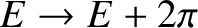be the angular velocity of precession of Mercury's eccentricity vector,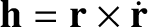. It follows that [see Equation (A.96)]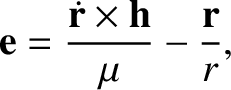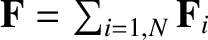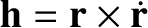(B.6)

which can be inverted to give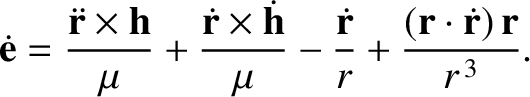(B.7)

Here, we have assumed, without loss of generality, thatis normal to(because any parallel component ofwould have no effect on the time evolution of).

Consider a Cartesian coordinate system,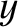,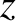that is instantaneously aligned with the orbit of Mercury, in such a manner that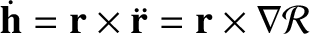and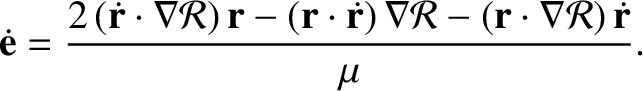. Thus, the orbital plane coincides with the-plane, and the perihelion lies (instantaneously) on the-axis. Because the eccentricity vector is always directed toward Mercury's perihelion, the rate of perihelion precession is equivalent to the component ofthat is normal to the orbital plane. In other words,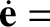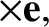(B.8)

Note that any component ofthat lies in the orbital plane would cause the orientation of this plane to evolve in time. In the following, we shall neglect this effect because it has a negligible influence on Mercury's perihelion precession rate (given Mercury's small orbital eccentricity, as well as its small orbital inclination with respect to the orbits of the other planets in the solar system.)

Let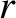,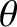,be a cylindrical coordinate system in the (,,) frame. Mercury's orbital plane thus corresponds to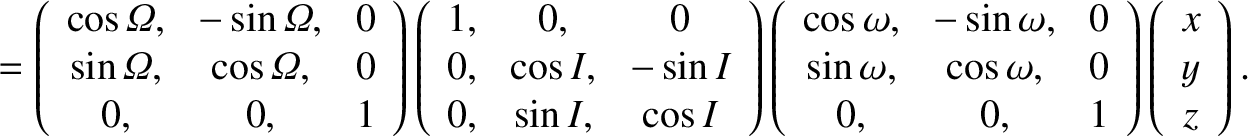. Moreover, we can write (see Section 4.4)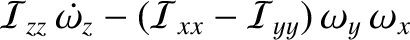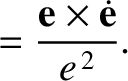(B.9) and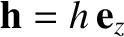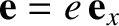(B.10)

as well as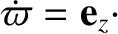(B.11)

where the Cartesian components of the unit vectors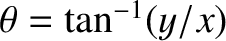,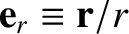, and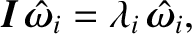are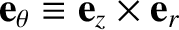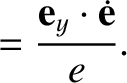(B.12)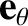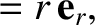(B.13) and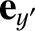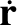(B.14)

Furthermore,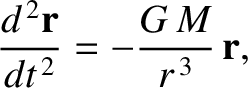(B.15)

whereis Mercury's orbital major radius. (See Section 4.8.) Incidentally, the angleis Mercury's true anomaly. (See Section 4.11.). It follows from Equations (B.5) and (B.9)-(B.11) that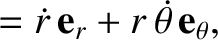(B.16)

Moreover, Equations (B.8) and (B.12)-(B.13) yield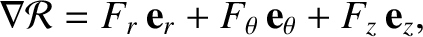(B.17)

However, differentiation of Equation (B.16) with respect to time gives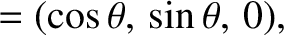(B.18)

Thus, we obtain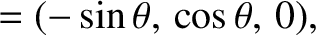(B.19)

Finally, from Equation (B.11),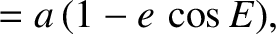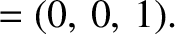(B.20) and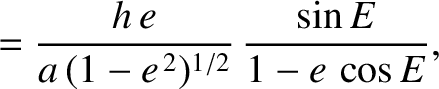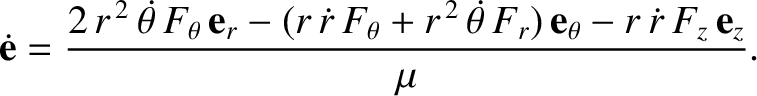(B.21)

which yields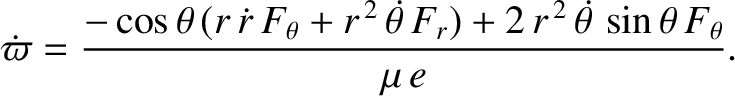(B.22)Next: Evaluation of disturbing function Up: Perihelion precession of Mercury Previous: Time evolution of eccentricity
Richard Fitzpatrick 2016-03-31